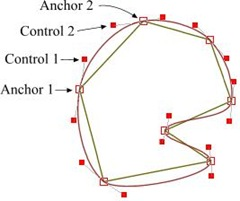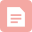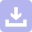# 穿过已知点画平滑曲线（3次贝塞尔曲线）

http://liyiwen.javaeye.com/blog/705489  。看到一种比较好的方法：（c++版，其它语言只要把数组和可变数组稍微变一下就能用）

### 翻译] AGG 之贝塞尔插值

Interpolation with Bezier Curves  贝塞尔插值

A very simple method of smoothing polygons 一种非常简单的多边形平滑方法但我有个大胆的推测，我觉得肯定还存在更简单的方法。比如，使用三次贝塞曲线(cubic Bezier)进行近似。贝塞尔曲线有两个固定点（起点和终点），另加两个决定曲线形状的控制点(CP）。关于贝塞尔曲线的更多知识可以在搜索引擎中找到，比如，你可以参考 Paul Bourke 的站点 。 现在给贝塞尔曲线的锚点（固定点），也就是多边形的某一对顶点，那么问题是，我们怎么计算控制点的位置？我运行 Xara X 然后画出了右边这个图形，这很简单，所以我决定尝试下计算出它们的坐标。很显然，多边形两条相邻边的两个控制点与这两个控制点之间的顶点应该在一条直线 上，只有这样，两条相邻的插值曲线才能平滑地连接在一起。所以，这两个控制点应该是相对于顶点是对称的，不过，也不全是……，因为真正的对称就要求它们与 中心点的距离应该是相等的，但对于我们的情况中并不完全是这样的。一开始，我试着先算出多边形两条边的角平分线，然后把控制点放在这条角平分线的垂直线 上。但从图上可以看到，控制点的连线并不会总是垂直于角平分线的。

This method is pure heuristic and empiric. It probably gives
a wrong result from the point of view of strict mathematical
modeling. But in practice the result is good enough and it
requires absolute minimum of calculations. Below is the source code
that has been used to generate the lions shown above. It's
not optimal and just an illustration. It calculates some variables
twice, while in real programs we can store and reuse them in the
// Assume we need to calculate the control
// points between (x1,y1) and (x2,y2).
// Then x0,y0 - the previous vertex,
//      x3,y3 - the next one.

double xc1 = (x0 + x1) / 2.0;
double yc1 = (y0 + y1) / 2.0;
double xc2 = (x1 + x2) / 2.0;
double yc2 = (y1 + y2) / 2.0;
double xc3 = (x2 + x3) / 2.0;
double yc3 = (y2 + y3) / 2.0;

double len1 = sqrt((x1-x0) * (x1-x0) + (y1-y0) * (y1-y0));
double len2 = sqrt((x2-x1) * (x2-x1) + (y2-y1) * (y2-y1));
double len3 = sqrt((x3-x2) * (x3-x2) + (y3-y2) * (y3-y2));

double k1 = len1 / (len1 + len2);
double k2 = len2 / (len2 + len3);

double xm1 = xc1 + (xc2 - xc1) * k1;
double ym1 = yc1 + (yc2 - yc1) * k1;

double xm2 = xc2 + (xc3 - xc2) * k2;
double ym2 = yc2 + (yc3 - yc2) * k2;

// Resulting control points. Here smooth_value is mentioned
// above coefficient K whose value should be in range [0...1].
ctrl1_x = xm1 + (xc2 - xm1) * smooth_value + x1 - xm1;
ctrl1_y = ym1 + (yc2 - ym1) * smooth_value + y1 - ym1;

ctrl2_x = xm2 + (xc2 - xm2) * smooth_value + x2 - xm2;
ctrl2_y = ym2 + (yc2 - ym2) * smooth_value + y2 - ym2;

consecutive steps.


// Number of intermediate points between two source ones,
// Actually, this value should be calculated in some way,
// Obviously, depending on the real length of the curve.
// But I don't know any elegant and fast solution for this
// problem.
#define NUM_STEPS 20

void curve4(Polygon* p,
double x1, double y1,   //Anchor1
double x2, double y2,   //Control1
double x3, double y3,   //Control2
double x4, double y4)   //Anchor2
{
double dx1 = x2 - x1;
double dy1 = y2 - y1;
double dx2 = x3 - x2;
double dy2 = y3 - y2;
double dx3 = x4 - x3;
double dy3 = y4 - y3;

double subdiv_step  = 1.0 / (NUM_STEPS + 1);
double subdiv_step2 = subdiv_step*subdiv_step;
double subdiv_step3 = subdiv_step*subdiv_step*subdiv_step;

double pre1 = 3.0 * subdiv_step;
double pre2 = 3.0 * subdiv_step2;
double pre4 = 6.0 * subdiv_step2;
double pre5 = 6.0 * subdiv_step3;

double tmp1x = x1 - x2 * 2.0 + x3;
double tmp1y = y1 - y2 * 2.0 + y3;

double tmp2x = (x2 - x3)*3.0 - x1 + x4;
double tmp2y = (y2 - y3)*3.0 - y1 + y4;

double fx = x1;
double fy = y1;

double dfx = (x2 - x1)*pre1 + tmp1x*pre2 + tmp2x*subdiv_step3;
double dfy = (y2 - y1)*pre1 + tmp1y*pre2 + tmp2y*subdiv_step3;

double ddfx = tmp1x*pre4 + tmp2x*pre5;
double ddfy = tmp1y*pre4 + tmp2y*pre5;

double dddfx = tmp2x*pre5;
double dddfy = tmp2y*pre5;

int step = NUM_STEPS;

// Suppose, we have some abstract object Polygon which
// has method AddVertex(x, y), similar to LineTo in
// many graphical APIs.
// Note, that the loop has only operation add!
while(step--)
{
fx   += dfx;
fy   += dfy;
dfx  += ddfx;
dfy  += ddfy;
ddfx += dddfx;
ddfy += dddfy;
}
p->AddVertex(x4, y4); // Last step must go exactly to x4, y4
}博客

09-126528博客

01-292万+下载

12-02博客

02-22574博客

11-032万+博客
CSS3 三次贝塞尔曲线(cubic-bezier)

10-243万+博客
openGL参数曲线-----三次贝塞尔曲线

03-06442博客
unity 控制点 贝塞尔曲线_求三次贝塞尔曲线的控制点
02-11585博客

01-13102博客

10-198591博客

09-161万+博客

10-08262博客
OpenGL - 三次Bezier曲线的绘制
10-186006博客
golang 绘图库_Golang 绘图基础 -绘制简单图形
12-242448博客
go-chart实现折线图/饼图/柱状图绘制
03-306404下载

04-08博客

11-27464博客

03-011767

### “相关推荐”对你有帮助么？

•非常没帮助
•没帮助
•一般
•有帮助
•非常有帮助点击重新获取扫码支付余额充值